# Which signal continues to reach AM or FM

## Frequency modulation

In addition to the older amplitude modulation, FM frequency modulation is another type of analog modulation that is of great importance in communications engineering. The information, the modulation signal, acts on the frequency of the carrier signal and leaves its amplitude unchanged. FM belongs to angle modulation and is closely related to phase modulation. The best-known area of ​​application for FM is VHF radio reception and in event technology it is wireless radio microphones.

If the information signal influences modules that determine the frequency in the carrier oscillator, a simple method for generating an FM is obtained. If it is a parallel resonant circuit of an LC oscillator, the capacitance can be supplemented by a capacitance diode (varicap) or a condenser microphone. Further possibilities arise with voltage-controlled sawtooth or square wave generators, which generate a synthetic sinusoidal signal with special diode circuits.

With a condenser microphone, the sound pressure changes the distance between the membranes in the capsule and the capacitance value varies accordingly. When modulating the pn junction of a capacitance diode, a blocking zone is maintained with a DC bias voltage. It has useful capacitive properties due to its charge distribution. An additional piece of information applied to this diode, the actual modulation signal, changes the width of the junction without breaking it down completely. The change in the capacitance value and, proportionally, the carrier frequency are determined directly from the information signal.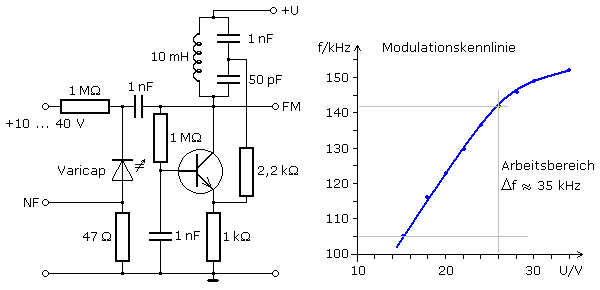The picture shows an oscillator circuit that works together with the Varicap as an FM modulator. There is a basic basic circuit, as the base has a direct ground reference for the signal via the 1 nF capacitor. The LC generator corresponds to a Colpitts circuit with subdivided C and the positive feedback branch via 2.2 kΩ to the emitter. If you recall the alternating current equivalent circuit, the potentials of the operating voltage and ground are identical in relation to the signal. The capacitance diode is consequently connected in parallel to the LC resonant circuit and has a say in the frequency of the oscillator.

The diagram shows the modulation characteristic that was recorded without an LF signal by changing the diode reverse voltage. The linear working range between 15 and 26 V, which can be used for modulation, corresponds to a frequency range of 35 kHz.

### The FM modulation signal

With a diode blocking voltage of 21 V, the oscillator or carrier frequency for the circuit shown above is around 125 kHz. If the entire linear characteristic range is to be used, the amplitude of the LF signal to be modulated can be a maximum of 5 V. A diode reverse voltage of 16 V remains for the positive peak value and 26 V for the negative peak value. The two associated stroke frequencies of 108 kHz and 142 kHz can be read from the characteristic curve.

A direct connection between the AF amplitude and the frequency deviation can be seen. When modulating audio signals, the volume determines the frequency deviation. The following graphic shows these relationships for a large and small AF signal amplitude. In the FM modulation signal, all frequencies between the two limit values ​​are passed through continuously. The unmodulated carrier frequency occurs in the low-frequency zero crossing.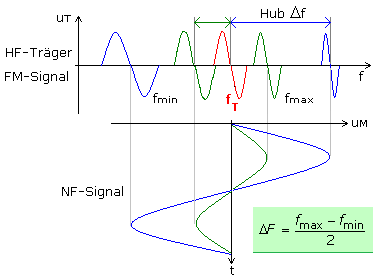For the following oscillogram images, unrealistic frequency ratios between HF and LF were chosen to show how an amplitude or frequency change of the LF signal is represented in the FM signal. The carrier frequency is constant. The modulation index η is proportional to the signal amplitude.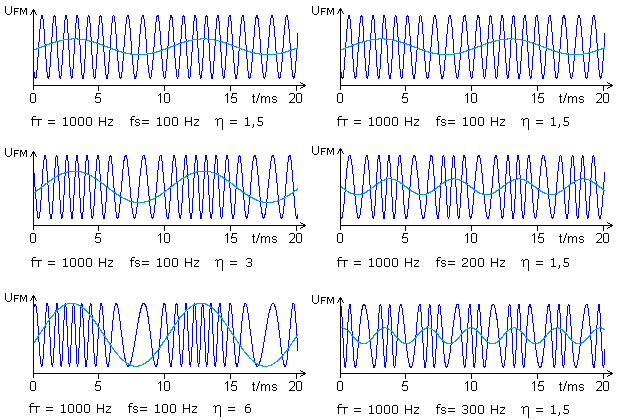The diagrams on the left show that the frequency deviation increases with increasing AF amplitude, represented by the light blue curve. The stroke ΔF is the difference between the highest and lowest instantaneous frequency in the FM signal. The highest frequencies in the FM signal are assigned to the range of the positive AF amplitude and the lowest value to the peak value of the negative signal half-wave. The diagrams on the right show that with a constant signal amplitude, the frequency of frequency changes between the maximum and minimum instantaneous frequency in the FM signal depends directly on the LF frequency. With a constant frequency change between the two corner values, the FM signal cannot be described by sine or cosine functions.

The amplitude of the signal voltage, the volume, is directly proportional to the frequency deviation of the FM signal.
The signal frequency, the pitch, determines the frequency of the change between the maximum and minimum instantaneous frequency of the FM signal.

### Frequency deviation

In the FM signal, a frequency range that is symmetrical to the carrier frequency is passed through. The frequency deviation is calculated with the two corner values ​​of the frequency. It can be specified as the peak deviation, which can be clearly measured in the case of a square-wave LF signal, since only the two corner frequencies occur in the FM signal. In the case of sinusoidal signals, the effective stroke can also be specified, which is smaller by a factor of √2.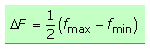### Degree of modulation

In analogy to amplitude modulation, a degree of modulation can also be defined for FM. It is to be understood as a measure of the relative change in the carrier signal fT. The degree of modulation has no practical significance and must not be confused with the important modulation index.### Modulation index

The relationship between the frequency deviation and the signal frequency is called the modulation index and is a characteristic variable in frequency modulation. As shown above, the modulation index is proportional to the AF amplitude. The code letter η, Greek eta, is used to differentiate between the degree of modulation.### Bandwidth

The following Fourier spectra show that the bandwidth of an FM signal can be very large. In any case, it is greater than twice the frequency deviation. In practice, one reckons with a finite bandwidth. All external spectral components of the FM spectrum with amplitudes less than 10% of the maximum amplitude are not taken into account. For FM stereo signal transmission in the VHF broadcasting range, the bandwidth must be calculated according to equation 2. With it, spectral components smaller than 5% are neglected so that the distortion factor in the received signal remains below 1%.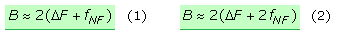In VHF radio (mono) the hub is 75 kHz and LF signals with a maximum of 15 kHz are transmitted. The transmitter bandwidth is calculated according to equation 1 to 180 kHz and according to equation 2 to 210 kHz. A comparable amplitude modulation would only require a bandwidth of 30 kHz. With modulation indices above 1, a frequency-modulated signal has a much higher bandwidth than a comparable amplitude modulation. Another page deals with FM stereo broadcasting on the VHF broadcast band.

### FM Fourier spectra

The spectra are the result of the Fourier analysis of a simulation program with an internal FM source in which the frequencies of the carrier and the signal as well as the modulation index were freely adjustable.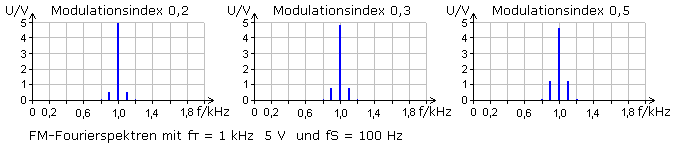With a small modulation index η ≤ 0.5, the spectrum is similar to that of amplitude modulation. Symmetrical to the carrier line there are two spectral lines at a distance of the signal or modulation frequency. This frequency spacing does not correspond to the frequency deviation, which remains the same with a constant signal frequency. The LF frequency line is not available. In contrast to AM, the amplitude of the carrier changes with the modulation index. With these small modulation indices, the value for η can be calculated from the ratio of the amplitude sum of the side lines to the carrier line.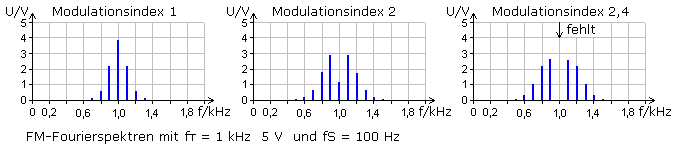With larger modulation indices from η> 0.5, further side frequencies occur symmetrically to the carrier frequency. They are at fixed intervals of ± 2 · fNf, ± 3 · fNf ... ± n · fNf and are called higher-order side frequencies. Their distance from one another is equal to the signal frequency. The frequency deviation cannot be read off in the spectrum here either. The amplitudes of the side lines vary depending on the modulation index. At η = 2.4 the amplitude of the carrier line has the value zero and is no longer present in the FM signal. The modulation index cannot be determined from the spectrum. The bandwidth of the FM signal increases as the modulation index increases.If the LF signal frequency is varied with a constant modulation index, then it only determines the distance between the frequency lines appearing in the spectrum. The amplitudes of the spectral lines remain constant.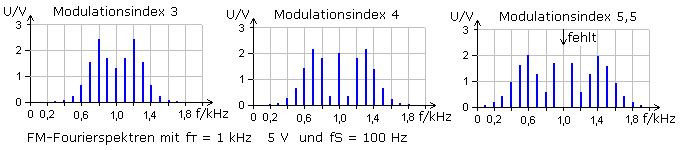If the modulation index is increased with a constant LF signal frequency, the number of side lines of higher order increases. The amplitudes change, but are smaller overall. The carrier line is missing again at η = 5.5. The modulation frequencies selected in this simulation no longer permit higher modulation indices for an undistorted FM signal.

The two stroke frequencies fmin = fT - ΔF and fmax = fT + ΔF can only occur in the spectrum if they happen to be an integral multiple of the information frequency.

### Bessel functions and FM spectrum

The very different amplitude behavior of the carrier and the side frequency pairs as a function of the modulation index results from the course of the Bessel functions. Understanding them requires knowledge of higher mathematics and the solution of differential equations, therefore only the result and the interaction with the frequency spectra of the FM are shown here.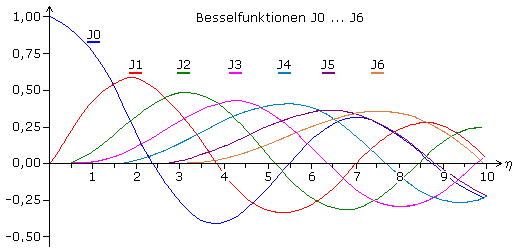The amplitude value of the carrier frequency depending on the modulation index can be read from the course of the J0 curve. At η = 2.4, the carrier line is no longer included in the FM signal for the first time. It then reappears, returning to zero at 5.5 and later at 8.65.

The higher functions from J1 onwards determine the amplitude values ​​of the corresponding pairs of side lines as a function of the modulation index. If the Bessel functions are negative, the amplitude values ​​of the side line pair in the Fourier spectrum continue to be shown as positive. If the phase diagram were also displayed, a phase shift of 180 ° would appear there.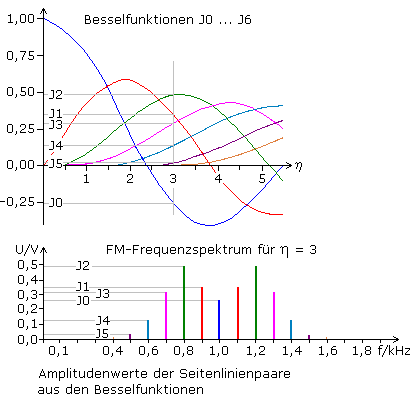The diagram shows the relationship between the Bessel functions and an FM spectrum. The spectrum was created in the simulation program with a carrier frequency of 1 kHz and an amplitude of 1 V. The modulation took place with a sinusoidal signal with f = 100 Hz with a modulation index η = 3.

### Mathematical background to FM

The timing diagram of an AM signal shows that the information lies in the changes in amplitude of the carrier. If the time diagram of an FM signal is also interpreted, the information is contained in the changes in the carrier frequency, i.e. ultimately in the zero crossings of the FM signal. If the zero crossings of the carrier oscillation change, this can also be understood as a phase shift or phase angle change. As mentioned above, the frequency modulation counts to the angle modulation.

If in the case of the unmodulated carrier oscillation, i.e. with a constant angular frequency, the angle is represented as a function of time φ = ω · t, a linear increase is obtained. With ω = 2 · π · f there is another linear relationship between the phase angle and the frequency φ = 2 · π · t · f. The figure shows the time diagram of the phase angle for three frequencies.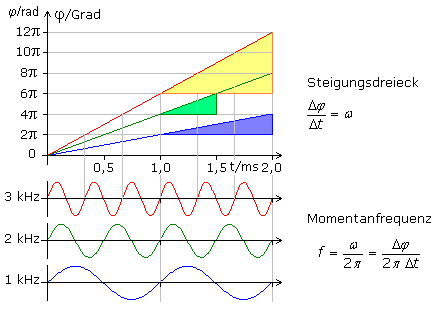The highlighted slope triangles stand for the rate of change of the phase angle. When dividing by 2 · π, the instantaneous frequency follows. Every FM signal has a non-linear phase curve. The rates of change and the associated instantaneous frequencies can be determined as above, with the differential quantity dφ / dt being mathematically more precise.

The following figure shows, in a simplified manner, the time dependency of the phase angle in the FM signal. The phase angle curve of the unmodulated carrier (blue) is linear. This carrier is modulated with a cosine signal of 0.5 kHz. For better clarity, the signal amplitude was quantized in three stages. The positive AF peak value leads to 1.5 kHz in the FM signal and the negative peak value to 0.5 kHz. In between, the 1 kHz carrier remains unmodulated. The resulting FM signal can be seen in the timing diagram and the modulating information signal with the quantization levels is shown below it.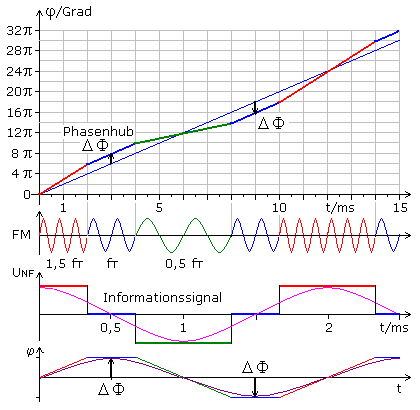For two time units (0 ... 2) ms, the FM signal shows 1.5 kHz, with three full periods corresponding to the radian measure 6π being passed through. Then the NF changes suddenly and for a further two time units (2 ... 4) ms, 4π corresponding to the carrier frequency of 1 kHz are passed through. At 4 ms, the modulation signal changes abruptly again and the FM shows the lowest instantaneous frequency for four time units (up to 8 ms) at 0.5 kHz. During this time, two periods equal to 4π are passed through. The unmodulated carrier frequency then occurs again for two time units. From 10 ms, the frequency jumps to the highest instantaneous frequency for a total of four time units, with six periods being run through, a radian of 12π.

The last time diagram shows the dependency of the phase angle in the FM signal reduced to the trapezoid shape. The phase angle of the FM signal deviates from the mean value of the unmodulated carrier by the maximum phase deviation ΔΦ. The cosine-shaped information signal leads to a sinusoidal phase deviation.

With a sinusoidal LF modulation voltage, the sinusoidal phase deviation signal lags behind by 90 °.

If the amplitude is increased at the same AF frequency, the upper corner frequency increases and the lower frequency decreases in the FM signal, since the frequency deviation increases. The phase deviation also increases, since a higher number of periods per time unit and a smaller number of periods are passed through in the FM signal with the minimum frequency.

The maximum frequency deviation is proportional to the maximum phase deviation. ΔF ~ ΔΦ

If the frequency of the modulating LF signal is changed while the amplitude is constant, this affects the phase deviation. The dependency can be constructed graphically quite easily with quantized LF signals. The two NF frequencies differ by a factor of 2. The phase deviation is greater at the lower frequency.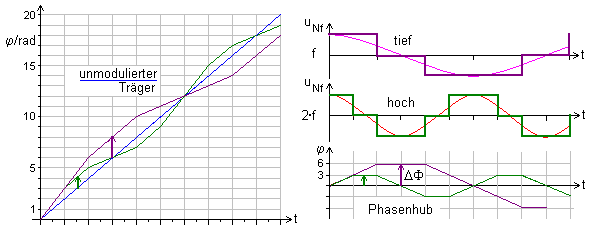The maximum phase deviation is inversely proportional to the modulation frequency. ΔΦ ~ 1 / fNf
The maximum phase deviation and the modulation index are the same. η = ΔF / fNf

The carrier frequency is modulated with a cosine signal and leads to a sinusoidal phase angle change. It is calculated from the time integral over the angular frequency. The amplitude of the change in angle, the phase deviation, is inversely proportional to the angular frequency. The time function of an FM signal then follows, written in mathematical equations: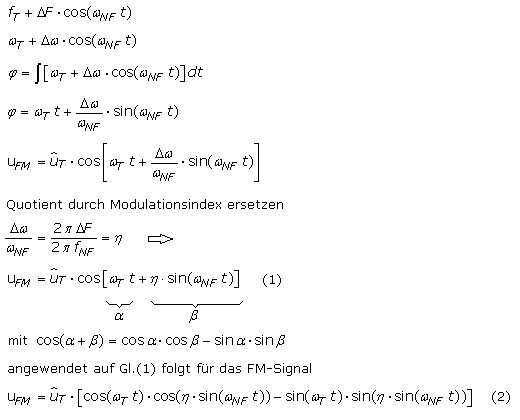Products of various angle functions appear in the expression in brackets. A first approach to a solution is found under the assumption that the modulation index is η «1. Some of these trigonometric functions are simplified and a trigonometric relationship can be applied to the rest.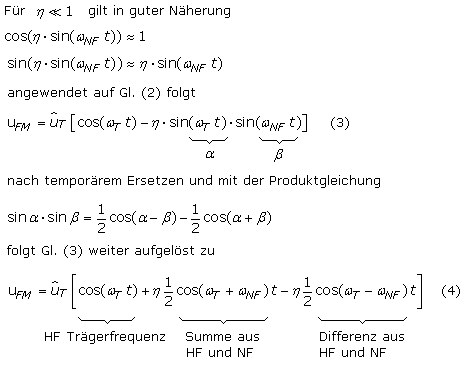With a very small modulation index, the FM signal is composed of the three marked cosine signals. This agrees with the Fourier spectra shown at the beginning, which are similar to an AM spectrum. The modulation index is in the equation here. The negative sign in the differential expression indicates a phase shift of 90 ° in relation to the carrier.

The Bessel functions must be used for the calculation for any modulation index. According to the specialist literature "Modulationsverfahren von E. Stadler", the time functions can be written as shown below, whereby the amplitudes Jo (η) ... Jn (η) of the spectral components are given by the Bessel functions: